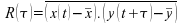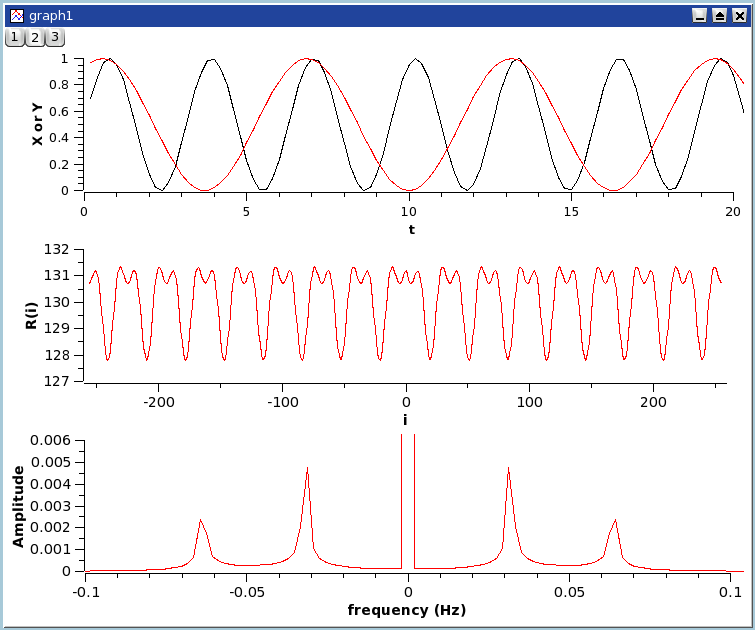# Correlation

This function is accessed with the Correlate command. It is located in the Analysis Menu whenever a table is selected. The correlation function, also known as the covariance function, is used to test the similarity of two signals x(t) and y(t). It is computed as:

Equation 6-2. Covariance function of two signals x(t) and y(t)in whichandare the mean values of the signals x(t) and y(t), respectively.

If the number of points is N, the function will be computed between -N/2 and N/2. The abscissae are therefore point indexes and not t values.

Figure 6-2. An example of a correlation between two sine functions.

The first plot shows the two signals, the second one is the correlation function between the two signal which shows that there are correlations, and the third one is the Fourier transform which is done to extract the characteristic frequencies of the correlation function.The correlation of a signal with itself can also be performed and is frequently used in spectral analysis (it is then called autocorrelation or autocovariance function).